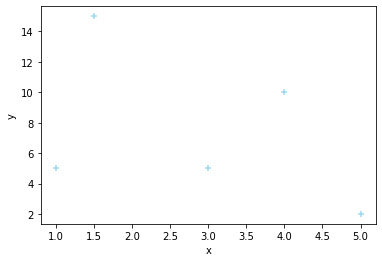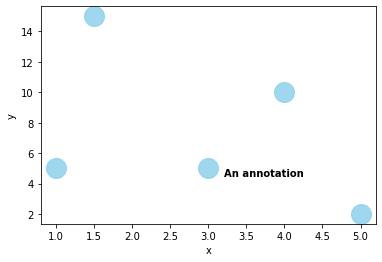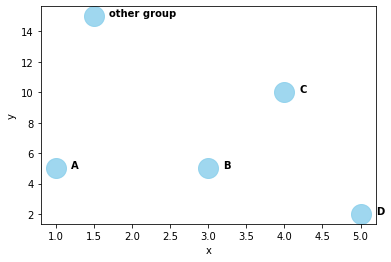## Basic scatterplot

You can create a basic scatterplot using `regplot()` function of seaborn library. The following parameters should be provided:

• `data` : dataset
• `x` : positions of points on the X axis
• `y` : positions of points on the Y axis
• `fit_reg` : if True, show the linear regression fit line
• `marker` : marker shape
• `color` : the color of markers
``````import pandas as pd
import numpy as np
import matplotlib.pylab as plt
import seaborn as sns

# Create dataframe
df = pd.DataFrame({
'x': [1, 1.5, 3, 4, 5],
'y': [5, 15, 5, 10, 2],
'group': ['A','other group','B','C','D']
})

sns.regplot(data=df, x="x", y="y", fit_reg=False, marker="+", color="skyblue")

plt.show()``````Once you have created the dataset and plotted the scatterplot with the previous code, you can use `text()` function of matplotlib to add annotation. The following parameters should be provided:

• `x` : the position to place the text in x axis
• `y` : the position to place the text in y axis
• `s`: the text

You can also specify the additional parameters such as `horizontalalignment`, `size`, `color`, `weight` to design your text.

``````# basic plot
sns.regplot(data=df, x="x", y="y", fit_reg=False, marker="o", color="skyblue", scatter_kws={'s':400})

plt.text(3+0.2, 4.5, "An annotation", horizontalalignment='left', size='medium', color='black', weight='semibold')

plt.show()``````## Use a loop to annotate each marker

If you want to annotate every markers, it is practical to use a loop as follow:

``````# basic plot
sns.regplot(data=df, x="x", y="y", fit_reg=False, marker="o", color="skyblue", scatter_kws={'s':400})

# add annotations one by one with a loop
for line in range(0,df.shape):
plt.text(df.x[line]+0.2, df.y[line], df.group[line], horizontalalignment='left', size='medium', color='black', weight='semibold')

plt.show()``````## Contact & Edit

👋 This document is a work by Yan Holtz. You can contribute on github, send me a feedback on twitter or subscribe to the newsletter to know when new examples are published! 🔥